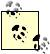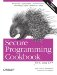# Generating Random Numbers

Problem

You want to generate some random floating-point numbers in the interval of [0.0, 1.0) with a uniform distribution.

Solution

The C++ standard provides the C runtime function rand in the header that returns a random number in the range of 0 to RAND_MAX inclusive. The RAND_MAX macro represents the highest value returnable by the rand function. A demonstration of using rand to generate random floating-point numbers is shown in Example 11-11.

Example 11-11. Generating random numbers using rand

```#include
#include
#include

using namespace std;

double doubleRand( ) {
return double(rand( )) / (double(RAND_MAX) + 1.0);
}

int main( ) {
srand(static_cast(clock( )));
cout << "expect 5 numbers within the interval [0.0, 1.0)" << endl;
for (int i=0; i < 5; i++) {
cout << doubleRand( ) << "
";
}
cout << endl;
}```

The program in Example 11-11 should produce output similar to:

```expect 5 numbers within the interval [0.0, 1.0)
0.010437
0.740997
0.34906
0.369293
0.544373```

Discussion

To be precise, random number generation functions, including rand, return pseudo-random numbers as opposed to truly random numbers, so whenever I say random, I actually mean pseudo-random.

Before using the rand function you need to seed (i.e., initialize) the random number generator with a call to srand. This assures that subsequent calls to rand won't produce the same sequence of numbers each time the program is run. The simplest way to seed the random number generator is to pass the result from a call to clock from the header as an unsigned int. Reseeding a random number generator causes number generation to be less random.

The rand function is limited in many ways. To begin with, it only generates integers, and only does so using a uniform distribution. Furthermore, the specific random number generation algorithm used is implementation specific and, thus, random number sequences are not reproducible from system to system given the same seed. This is a problem for certain kinds of applications, as well as when testing and debugging.

A much more sophisticated alternative to rand is the Boost Random library by Jens Maurer that has inspired the random number facilities proposed for TR1.TR1 stands for Technical Report One, and is an official proposed extension to the C++ 98 standard library.

The Boost Random library provides several high-quality random number generation functions for both integer and floating-point types, and support for numerous kinds of distributions. Example 11-12 demonstrates how you can produce random floating-point numbers in the interval [0,1).

Example 11-12. Using the Boost Random library

```#include
#include
#include

using namespace std;
using namespace boost;

typedef boost::mt19937 BaseGenerator;
typedef boost::uniform_real Distribution;
typedef boost::variate_generator Generator;

double boostDoubleRand( ) {
static BaseGenerator base;
static Distribution dist;
static Generator rng(base, dist);
return rng( );
}

int main( ) {
cout << "expect 5 numbers within the interval [0,1)" << endl;
for (int i=0; i < 5; i++) {
cout << boostDoubleRand( ) << "
";
}
cout << endl;
}```

The main advantage of the Boost Random library, is that the pseudo-random number generation algorithm has guaranteed and reproducible randomness properties based on the precise algorithm chosen. In Example 11-12 I use the Mersenne Twister generator (mt19937) because it offers a good blend of performance and randomness.Secure Programming Cookbook for C and C++: Recipes for Cryptography, Authentication, Input Validation & More
ISBN: 0596003943
EAN: 2147483647
Year: 2006
Pages: 241